Chapter 12, Problem 4PS

Chapter
Section
Textbook Problem

The unit cell of silicon carbide, SiC, is illustrated below. (a) In what type of unit cell are the (dark gray) C atoms arranged? (b) If one edge of the silicon carbide unit cell is 436.0 pm, what is the calculated density of this compound?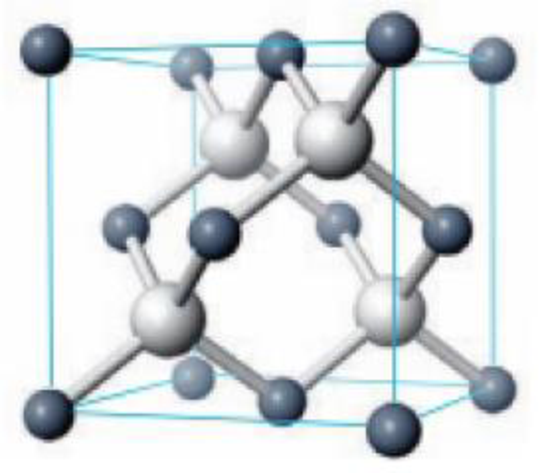A portion of the solid-state structure of silicon carbide.

(a)

Interpretation Introduction

Interpretation:

Type of unit cell where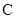atoms are arranged has to be calculated.

Concept introduction:

The face-centered cubic arrangement, the FCC atoms are located at each of the corners and the centers of all the cube faces.

Explanation

The unit cell for the

(b)

Interpretation Introduction

Interpretation:

The density of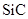has to be calculated.

Concept introduction:

The volume of the atom is given below,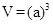The density of the unit cell is calculated as follows,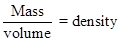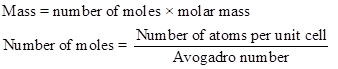Still sussing out bartleby?

Check out a sample textbook solution.

See a sample solution

The Solution to Your Study Problems

Bartleby provides explanations to thousands of textbook problems written by our experts, many with advanced degrees!

Get Started

Athletes should avoid frequent between-meal snacks. T F

Nutrition: Concepts and Controversies - Standalone book (MindTap Course List)

What is hereditarianism, and what is the invalid assumption it makes?

Human Heredity: Principles and Issues (MindTap Course List)

Why is there a lower limit to the mass of a main-sequence star?

Horizons: Exploring the Universe (MindTap Course List)

Which class has the largest individuals? Which is the most economically important?

Oceanography: An Invitation To Marine Science, Loose-leaf Versin